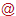Leibniz Institute for Plasma Science and Technology
Felix-Hausdorff-Str. 2
17489 Greifswald
GERMANY

https://www.inp-greifswald.de/en/
welcomeinp-greifswald [punkt] de

The Leibniz Institute for Plasma Science and Technology (INP) is the largest non-university institute in the field of low temperature plasmas, their basics and technical applications in Europe. The institute carries out research and development from idea to prototype. The topics focus on the needs of the market. At present, plasmas for materials and energy as well as for environment and health are the focus of interest.

## Streamer-surface interaction in an atmospheric pressure dielectric barrier discharge in argon - dataset

The results of the modelling of an atmospheric-pressure dielectric barrier discharge (DBD) in argon obtained using a time-dependent and spatially two-dimensional fluid-Poisson model in axisymmetric geometry are provided in this dataset. The spatiotemporal evolution of the electron number density, electric current, applied, memory and gap voltage, the spatial distribution of the number density of electrons and argon excited atoms, the electric field, electron production and loss rates, and surface charge density distribution are used for the analysis of the surface-streamer interaction and the cathode-layer formation. The analyses showed that the radial deflection of the volume streamer is driven by the free electrons that remained in the volume from the Townsend pre-phase and guided by the radial component of the electric field. The surface discharge, occurring simultaneously with the propagation of the deflected volume streamer, is governed by ion-induced secondary electron emission and the surface charges accumulated on the dielectric.

FieldValue
Group
Authors
Release Date
2022-01-24
Identifier
0e609bc7-3a55-4b13-95ca-a0f9d5b2813a
Permanent Identifier (DOI)
Permanent Identifier (URI)
Is supplementing
Plasma Source Name
Plasma Source Application
Plasma Source Specification
Plasma Source Properties

The object of interest is streamer-surface interaction and the cathode-layer formation during the first discharge event in the single-filament dielectric barrier discharge (DBD) in argon at atmospheric pressure. The modelling is performed for the DBD in an asymmetric arrangement with a 1.5 mm gap, electrode radius of 2 mm and 0.5 mm thick dielectric (alumina, relative permittivity 9) covering grounded electrode, driven by sinusoidal voltage (voltage amplitude of 3 kV and frequency 60 kHz).

Plasma Medium Name
Plasma Medium Properties

Pure argon, gas pressure is 760 Torr, constant gas temperature of 300 K

Plasma Diagnostics Name
Plasma Diagnostics Properties

Model: Fluid-Poisson model in cylindrical geometry;
Computational software: COMSOL Multiphysics® v. 5.6 (www.comsol.com, COMSOL AB, Stockholm, Sweden);
Numerical method: Finite element method;
Element type: Linear Lagrange elements for particle balance equations, quadratic Lagrange elements for Poisson equation, linear discontinuous Lagrange elements for the surface charge density balance equation;
Time-stepping: Backward differentiation formula (BDF), with automatically adapted time-step size and the BDF order (in the range from 1 to 5);
Solver: Fully coupled constant Newton solver, with the direct linear solver (PARDISO);
Tolerance: 0.0001
Mesh: Remeshing is done to reduce calculation time, where the continuation of the calculations from the last calculated time step is done after each remeshing. The whole presented time range is calculated with four remeshings:
1) t: [0, 3.9] microseconds, number of mesh elements: 360194, DoF: 3340893;
2) t: [3.9, 3.94793] microseconds, number of mesh elements: 360194, DoF: 3340893;
3) t: [3.94793, 3.955] microseconds, number of mesh elements: 401562, DoF: 3726070;
4) t: [3.955, 4] microseconds, number of mesh elements: 572190, DoF: 5172073;
5) t: [4, 4.03] microseconds, number of mesh elements: 464810, DoF: 4153330;
Calculated currents, surface charge density and the total charge for different time intervals were post-processed in Origin Pro (https://www.originlab.com/). The number densities, electric field and electron production rates were exported using LiveLink™ for MATLAB® (https://www.comsol.com/livelink-for-matlab) and post-processed using python3 (https://www.python.org) and Matplotlib (https://matplotlib.org).

Plasma Diagnostics Procedure

An atmospheric-pressure DBD in argon is investigated by means of time-dependent and spatially two-dimensional fluid-Poisson modelling in an axisymmetric geometry. The characteristic structure consisting of the volume streamer propagating just above the dielectric and simultaneous development of an additional surface discharge near the cathode during the initial discharge is observed and explained. The model comprises a set of balance equations for the particle number densities of electrons and the most important argon species (atomic Ar+ and molecular Ar2+ ions, as well as the lumped excited atomic Ar∗ and molecular Ar2∗ states of argon), Poisson’s equation for the electric potential and field, the electron energy balance equation and a balance equation for the surface charge density. The quasi-neutral initial conditions and physically-based boundary conditions, accounting for thermal flux and partial reflection of the particles, are used. The additional term describing the ion-induced emission of secondary electrons is included into the boundary conditions for the balance equations for the particle number density and energy density of electrons.

Language
English